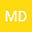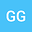A structurally damped σ-evolution equation with nonlinear memory
••• Marcello D'Abbicco,
• Giovanni Girardi
Marcello D'Abbicco
University of Bari

Corresponding Author:m.dabbicco@gmail.com

Author ProfileGiovanni Girardi
Università degli Studi di Bari Aldo Moro
Author Profile## Abstract

In this paper we investigate the global existence of small data solutions for the following structurally damped σ-evolution model with nonlinear memory term % $u_{tt}+(-\Delta)^\sigma u+\mu(-\Delta)^{\frac{\sigma}{2}}u_t=\int_0^t (1+\tau)^{-\gamma}|u_t(\tau,\cdot)|^p\,d\tau,$ % with σ>0. In particular, for $\gamma\in ((n-\sigma)/n,1)$ we find the sharp critical exponent, under the assumption of small data in~$L^1$. Dropping the~$L^1$ smallness assumption of initial data, we show how the critical exponent is consequently modified for the problem. In particular, we obtain a new interplay between the fractional order of integration~$1-\gamma$ in the nonlinear memory term, and the assumption that initial data are small in~$L^m$, for some~$m>1$.
01 Feb 2020Submitted to Mathematical Methods in the Applied Sciences
07 Feb 2020Submission Checks Completed
07 Feb 2020Assigned to Editor
21 Feb 2020Reviewer(s) Assigned
12 May 2020Review(s) Completed, Editorial Evaluation Pending
17 May 2020Editorial Decision: Revise Minor